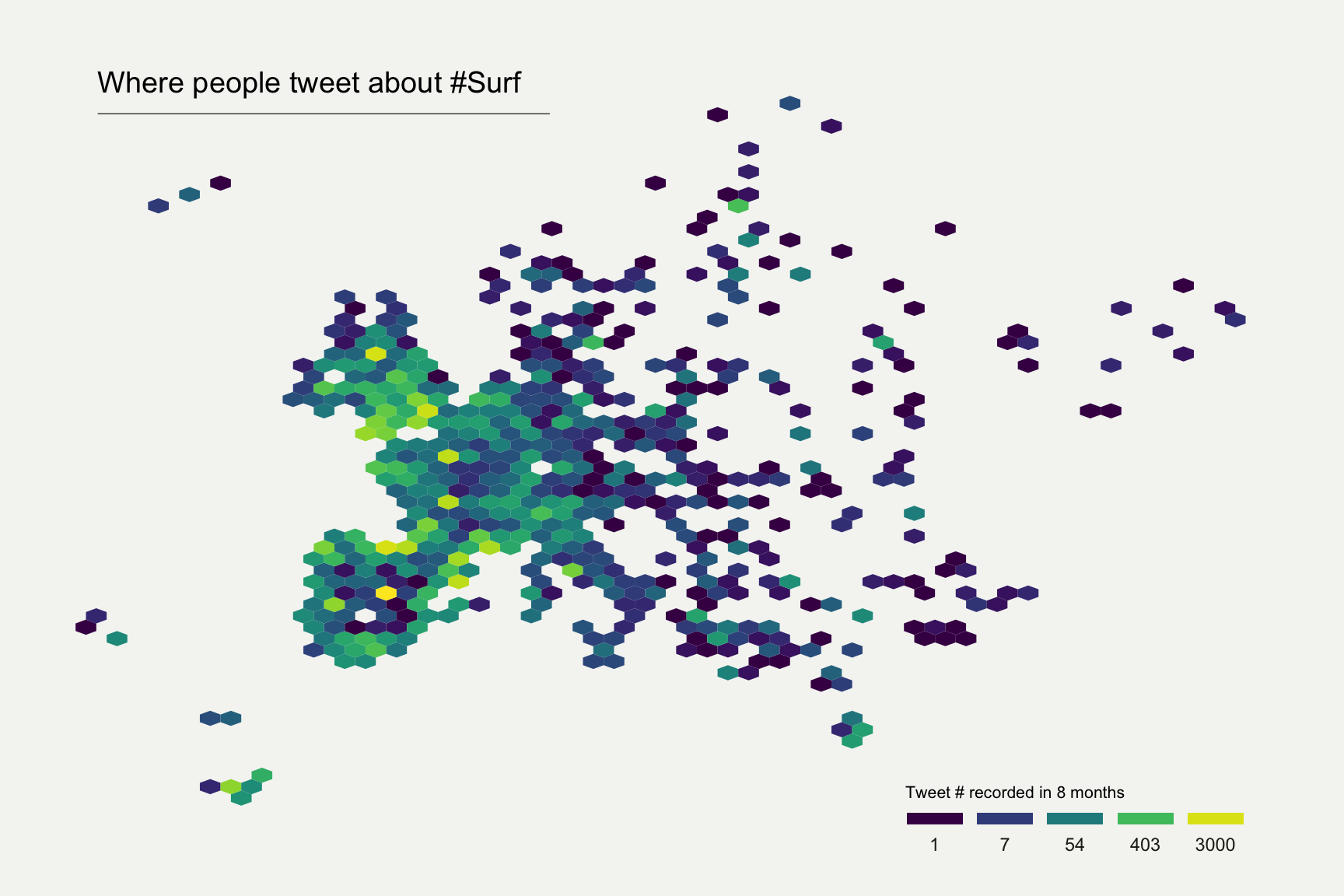# Definition

The term `hexbin map` refers to two different concepts:

• The first definition is based on an unusual geospatial object where all regions of the map are represented as hexagons. Here is an example representing the US states, each represented as an hexagon. Note that here the data input provides `one value per state` and a `specific shape file` with hexagone boundaries.
``````# library
library(tidyverse)

# Hexbin available in the geojson format here: https://team.carto.com/u/andrew/tables/andrew.us_states_hexgrid/public/map. Download it and then:
library(rgdal)

# I need to 'fortify' the data to be able to show it with ggplot2 (we need a data frame format)
library(broom)
spdf_fortified <- tidy(spdf, region = "google_name")

# Calculate the centroid of each hexagon to add the label:
library(rgeos)
centers <- cbind.data.frame(data.frame(gCentroid(spdf, byid=TRUE), id=spdf@data\$iso3166_2))

# Found here: https://www.cdc.gov/nchs/nvss/marriage-divorce.html

# Merge geospatial and numerical information
spdf_fortified = spdf_fortified %>%
left_join(. , data, by=c("id"="state"))

# Prepare binning
spdf_fortified\$bin = cut( spdf_fortified\$y_2015 , breaks=c(seq(5,10), Inf), labels=c("5-6", "6-7", "7-8", "8-9", "9-10", "10+" ), include.lowest = TRUE )

# Prepare a color scale coming from the viridis color palette
library(viridis)
my_palette=rev(magma(8))[c(-1,-8)]

# plot
ggplot() +
geom_polygon(data = spdf_fortified, aes(fill = bin, x = long, y = lat, group = group) , size=0, alpha=0.9) +
geom_text(data=centers, aes(x=x, y=y, label=id), color="white", size=3, alpha=0.6) +
theme_void() +
scale_fill_manual(
values=my_palette,
name="Wedding per 1000 people in 2015",
guide = guide_legend( keyheight = unit(3, units = "mm"), keywidth=unit(12, units = "mm"), label.position = "bottom", title.position = 'top', nrow=1)
) +
ggtitle( "A map of marriage rates, state by state" ) +
theme(
legend.position = c(0.5, 0.9),
text = element_text(color = "#22211d"),
plot.background = element_rect(fill = "#f5f5f2", color = NA),
panel.background = element_rect(fill = "#f5f5f2", color = NA),
legend.background = element_rect(fill = "#f5f5f2", color = NA),
plot.title = element_text(size= 22, hjust=0.5, color = "#4e4d47", margin = margin(b = -0.1, t = 0.4, l = 2, unit = "cm")),
)``````• The second definition refers to the utilisation of 2D density techniques. Think of a scatterplot where the X axis is the longitude and the Y axis is the latitude. The graphic area is divided in a multitude of hexagones and the number of data point in each is counted and represented using a color gradient. Note that here `no map boundaries is needed`. It requires only a `list of latitude and longitude`.

``````# Libraries
library(tidyverse)
library(viridis)
library(hrbrthemes)
library(kableExtra)
options(knitr.table.format = "html")
library(mapdata)

# plot
data %>%
filter(homecontinent=='Europe') %>%
ggplot( aes(x=homelon, y=homelat)) +
geom_hex(bins=59) +
ggplot2::annotate("text", x = -27, y = 72, label="Where people tweet about #Surf", colour = "black", size=5, alpha=1, hjust=0) +
ggplot2::annotate("segment", x = -27, xend = 10, y = 70, yend = 70, colour = "black", size=0.2, alpha=1) +
theme_void() +
xlim(-30, 70) +
ylim(24, 72) +
scale_fill_viridis(
trans = "log",
breaks = c(1,7,54,403,3000),
name="Tweet # recorded in 8 months",
guide = guide_legend( keyheight = unit(2.5, units = "mm"), keywidth=unit(10, units = "mm"), label.position = "bottom", title.position = 'top', nrow=1)
)  +
ggtitle( "" ) +
theme(
legend.position = c(0.8, 0.09),
legend.title=element_text(color="black", size=8),
text = element_text(color = "#22211d"),
plot.background = element_rect(fill = "#f5f5f2", color = NA),
panel.background = element_rect(fill = "#f5f5f2", color = NA),
legend.background = element_rect(fill = "#f5f5f2", color = NA),
plot.title = element_text(size= 13, hjust=0.1, color = "#4e4d47", margin = margin(b = -0.1, t = 0.4, l = 2, unit = "cm")),
) ``````Note on the second map: This map shows the geographic position of about 200k tweets containing the hashtags `#surf`, `#windsurf` or `#kitesurf`. Read more about it here.

# What for

Hexbin or grid map has an `advantage` over usual choropleth maps. In choropleths, a large polygon’s data looks more emphasized just because of its size, what introduces a bias. Here with hexbin, each region is represented equally dismissing the bias.

There’s a `drawback` to this format though. Map readers generally recognize a geographic area by it’s shape and orientation to other areas. For instance, the geography of the US is well known and people easily identify different regions. In hexbin maps, these landmarks do not exist anymore what can confuse the audience. One solution for this is to choose a basemap that uses labels on top of your data layer.

# Variation

Hexbin map uses hexagons to split the area in several parts and attribute a color to it. Note that it is possible to use square instead of hexagones, resulting in a 2D histogram map.

# Common mistakes

All the mistakes presented in the choropleth section apply

# Related

The R and Python graph galleries are 2 websites providing hundreds of chart example, always providing the reproducible code. Click the button below to see how to build the chart you need with your favorite programing language.